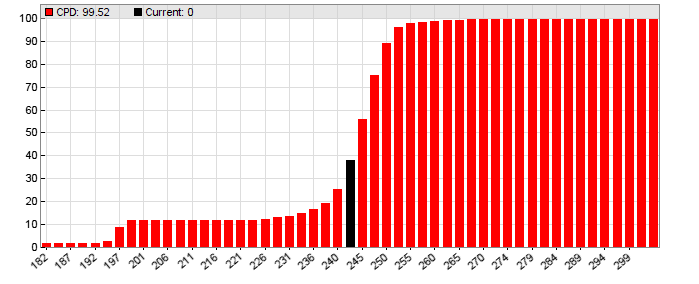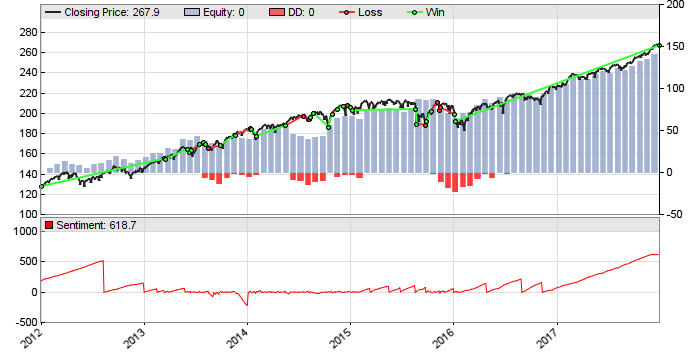# The Mechanical TurkWe can see thinking machines taking over more and more human tasks, such as car driving, Go playing, or financial trading. But sometimes it’s the other way around: humans take over jobs supposedly assigned to thinking machines. Such a job is commonly referred to as a Mechanical Turk in reminiscence to Kempelen’s famous chess machine from 1768. In our case, a Mechanical Turk is an automated trading algorithm based on human intelligence.

Theoretically, many trend trading systems would fall in the Turk category since they are based on following the herd. But here we’re not looking into trader’s opinions of the current market situation, but into their expectations of the future. There are several methods. The most usual is evaluating the Commitment Of Traders report, for which many platforms, also Zorro, provide easy-to-use indicators. But some publications (1) found that using the COT report for predicting the markets produces mixed results at best.

There’s a more precise way to get the market’s expectations. It’s using options premiums. If an option expires in 6 weeks, its current premium reflects what option buyers or sellers think about the underlying price in 6 weeks.

### The price probability distribution

For deriving the expected underlying price at expiration, we have to take all strike prices in consideration. Here’s the algorithm in short (I haven’t invented it, a longer description can be found in (2)). Assume SPY is currently trading at \$200. For getting the probability that it will rise to between 210 and 220 in 6 weeks, we’re looking into the 210 call and the 220 call. Suppose they trade at \$14 and \$10. So we can buy the 210 call and sell the 220 call and pay \$4 difference. If at expiration SPY is under 210, both contracts have no worth and we lose the 4 dollars. If it is above 220, our gain is the \$10 strike difference minus \$4 premium difference, so we win 6 dollars. If it is between 210 and 220, our win or loss is also inbetween, with an average of (-4+6)/2 = 1 dollar.

When options prices are “fair”, i.e. solely determined by probabilities where the price will end up at expiration, summing up over all possible outcomes yields zero profit or loss. So

-\$4×L + \$1×M + \$6×H = \$0

where L is the probability for SPY to end up below 210, M is the probability that it will be between 210 and 220, and H is the probability that it will be above 220. Since one of these three alternatives will always happen, the sum of all 3 probabilities must be 1:

L + M + H = 1

Now let’s assume that we know L already. Then we have two equations with two unknowns, which are easily solved:

5L – 5H = 1    =>  H = 0.2 + 0.2L
10L + 5M = 6    =>  M = 1.2 -2L

Assuming L = 50% yields H = 30% and M = 20%.

How do we now get the value L? We simply take the lowest strike offered at the market, and assume that the probability of SPY ending up even below that is zero. So our first L is 0.  We can now use the method above to calculate the M belonging to the interval between the lowest and the second-lowest strike. That M is then added to L since it’s the probability of SPY ending up at or below the second-lowest strike. We continue that process with the next interval, getting a specific L and M for any interval until we arrived at the highest strike. If the traders have been consistent with their assumptions, the final L – the sum over all Ms – should be now at or close to 1.

Here’s a small script that displays the 6-weeks L distribution of SPY:

```void main()
{
StartDate = 20170601;
LookBack = 0;

assetList("AssetsIB");
asset("SPY");

// load today's contract chain
contractUpdate(0,0,CALL|PUT);
printf("\n%i contracts",NumContracts);
if(!NumContracts) return;

// get underlying price
var Price,Current = priceClose(0);
printf("\nCurrent price %.2f",Current);

// plot CPD histogram
contractCPD(45);	// 6 weeks
int N = 0;
for(Price = 0.75*Current; Price < 1.25*Current; N++, Price += 0.01*Current)
plotBar("CPD",N,floor(Price),cpd(Price),BARS|LBL2,RED);
printf("\nExpected price %.2f",cpdv(50));

// compare with real future price
set(PEEK);
N = timeOffset(UTC,-45,0,0);
printf("\nFuture price %.2f",priceClose(N));
}```

The result is a histogram of expected probabilities, in percent, that the SPY price will be at or below the price at the x-axis six weeks in the future. I’ve marked the current price with a black bar.SPY 6 weeks price probability distribution at June 1, 2017

The contractCPD function generates the distribution with the above described algorithm, cpd returns the accumulated probability L (in percent) at a given price, and cpdv returns the price at a given L. Therefore, cpdv(50) is the median of market expectations. In our case, at June 1 2017, a modest price increase to \$245 was expected for mid-July (in fact the price ended up at \$245.51, but don’t get too excited – often trader’s expectations fall short by several dollars). We can also see an unusal step at the begin of the histogram: about 10% of traders expected a strong drop to below \$200. Maybe Trump twittered something that morning.

### The strategy

We will now exploit trader’s expectations for a strategy, and this way check if they have any merit. This is our mechanical Turk:

```#define Sentiment AssetVar

void run()
{
StartDate = 20120102;
EndDate = 20171231;
BarPeriod = 1440;

assetList("AssetsIB");
asset("SPY");
MaxLong = MaxShort = 1;

// load today's contract chain
contractUpdate(0,0,CALL|PUT);
int N = contractCPD(45);

// increase/decrease market sentiment
if(N) {
var Expect = cpdv(50) - priceClose();
if(Expect < 0)
Sentiment = min(Expect,Sentiment+Expect);
else
Sentiment = max(Expect,Sentiment+Expect);
}
if(Sentiment > 5)
enterLong();
else if(Sentiment < -5)
enterShort();

plot("Sentiment",Sentiment,NEW,RED);
}```

We’re checking the market expectation every day and add it to a previously defined Sentiment asset variable. If the expectation changes sign, so does Sentiment. If its amount has accumulated above 5, we buy a long or short position dependent on its sign. This way we’re only considering the market expectation when it’s either relatively strong or had the same direction for several days.

The result:The system produces about 20% annual return with profit factor 3. The red line in the lower chart is the Sentiment variable – we can see that it often steadily increases, but sometimes also decreases. Since SPY normally rises, shorting it with this strategy produces less profit than going long, but is still slightly profitable with an 1.16 profit factor.

### Predicting the price in 6 weeks

What are traders currently thinking of the SPY price in 6 weeks? For this, the first script above needs just be slightly modified so that it does not use historical options data, but connects to IB and downloads the current options chain with all prices:

```void main()
{
StartDate = NOW;
LookBack = 0;

assetList("AssetsIB");
asset("SPY");

// load today's contract chain
contractUpdate(0,0,CALL|PUT);
printf("\n%i contracts",NumContracts);
if(!NumContracts) return;

// get underlying price
var Price,Current = priceClose(0);
printf("\nCurrent price %.2f",Current);

// plot CPD histogram
printf("\nWait time approx %i minutes",1+NumContracts/200);
contractCPD(45);
int N = 0;
for(Price = 0.75*Current; Price < 1.25*Current; N++, Price += 0.01*Current)
plotBar("CPD",N,floor(Price),cpd(Price),BARS|LBL2,RED);
printf("\nExpected price %.2f",cpdv(50));
}```

This script must be started in Zorro’s Trade mode with the IB plugin selected. Mind the displayed “wait time”. IB sometimes needs up to ten seconds for returning the price of an option contract, so downloading the prices of all 6-weeks contracts can take half an hour or more.

I ran that script today (Dec 11 2018) and the option traders expect the SPY price to rise to \$269 in six weeks. So I’ll check the price by the end of January and post here if they have been right.

I’ve added the scripts to the 218 repository. You’ll need Zorro 1.99 or above, and SPY options EOD history for the first 2 scripts. You’ll really have to buy it this time, the free artificial options history won’t do for market sentiment.

### Conclusions

• A Turk can beat a thinking machine.
• Option traders tend to underestimate future price changes.
• But they are often right in the price direction.

## 11 thoughts on “The Mechanical Turk”

1.Alex says:

Great to have you back! I was missing your articles. Zorro is a beauty! Thank you. Are you on twitter?

2.Scott says:

Excellent and innovative approach!

What would you suggest investigating as the lowest cost way to build SPY option price history to test your strategy?

Thanks

3.jcl says:

Thank you. No, I’m not on Twitter, and for options price history we normally recommend iVolatility to clients.

4.NiloLion says:

Thank you so very much for sharing your knowledge with us. Thank you also for the fantastic work on Zorro! It’s absolutely marvelous to see you back…

5.Simone says:

Hello, very nice article as usual.

I’d like to share with you a doubt about this approach: the formula assumes the prices are “fair”, which might not be and this I believe can lead to a misbehaviour. Sticking to your example, the line “M = 1.2 -2L”, could lead to a negative M if L > 60% when the calculation algorithm gets to those 2 calls. I’ve taken an option chain from yahoo, put it in a spreadsheet and implemented the formulas explained in the article, and I get negative M here and there. How does contractCPD handle this? I can’t quite figure out a clever solution, I thought we could try to use a “starting L” > 0 so that no negative Ms occur, but there’s no such value in the chain I got.

Thanks,
Simone

6.jcl says:

Yes, since many effects influence option prices, the model is a bit simplistic. Some option buyers do not speculate on future prices at all, but buy options for insurance, and this way distort the price distribution. So the price distribution is no guarantee to reflect the real probabilities. The contractCPD function is more complex than described here, f.i. it calculates the probabilities from both ends at L=0 and L=1, and also uses an algorithm for fixing gaps or negative probabilities.

7.Simone says:

Thank you, I expected using both ends was likely to be the improvement for that.

8.Spekyl says:

Simone, would You like to share your file?

9.Tamas says:

Not sure what I am doing wrong, but I am getting a crash in what looks like the contractCPD function:

!SPY: 272.77000 0.03000 1
SPY: 0..8765
!Get Option Chain SPY-OPT–0–SMART–USD
Chain of 3243 SPY contracts
3243 contracts today
Current price 272.77
Wait time approx 17 minutes
Error 111: Crash in function: main() at bar 0
Logout.. ok

10.jcl says:

I don’t know either, but places where you can get help with coding or crashes are the Zorro forum or Zorro support.

This site uses Akismet to reduce spam. Learn how your comment data is processed.